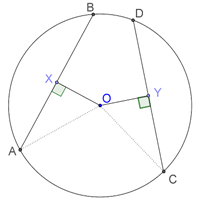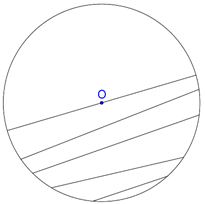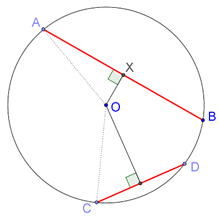# Equal and Unequal Chords

Equal and Unequal Chords

Now, we discuss some other fundamental results related to circles.

Theorem: Equal chords of a circle are equidistant from its center.

The following figure shows two equidistant chords AB and CD of a circle with center O. The distances of the two chords are respectively OX and OY:We need to show that OX = OY. We have joined OA and OC. Note that since OX is perpendicular to AB, it must also bisect AB. Similarly, since OY is perpendicular to CD, it must also bisect CD. Thus, since it is given that AB = CD, we have

$\raise.5ex\hbox{1}\kern-.1em/\kern-.15em\lower.25ex\hbox{2} {\rm{ }}\left( {AB} \right){\rm{ }} = {\rm{ }}AX{\rm{ }} = {\rm{ }}\raise.5ex\hbox{1}\kern-.1em/\kern-.15em\lower.25ex\hbox{2} {\rm{ }}\left( {CD} \right){\rm{ }} = {\rm{ }}CY$

Proof: Compare $$\Delta\mathrm O\mathrm A\mathrm X$$ with $$\Delta\mathrm O\mathrm C\mathrm Y$$.

1. OA = OC (radii of the same circle)

2. AX = CY (we just proved this above)

3. $$\angle {\rm{OXA }} = \angle {\rm{OYC }} = {\rm{ }}{90^0}$$

By the RHS criterion, $$\Delta {\rm{OAX}} \equiv \Delta {\rm{OCY}}$$. Thus, OX = OY, which means that AB and CD are equidistant from O.

The converse theorem also holds.

Converse: Two chords of a circle which are equidistant from its center must have the same length.

Proof: Referring to the previous figure again, we compare $$\Delta\mathrm{OAX}$$ with $$\Delta\mathrm{OCY}$$

1. OA = OC (radii of the same circle)

2. OX = OY (given)

3. $$\angle {\rm{OXA }} = \angle {\rm{OYC }} = {\rm{ }}{90^0}$$

By the RHS criterion again, the two triangles are congruent, and hence AX = CY. Since OX and OY must also bisect AB and CD respectively (why?), we have

${\rm{AB }} = {\rm{ }}2{\rm{AX }} = {\rm{ }}2{\rm{AY }} = {\rm{ CD}}$

Thus, the two chords are of equal length.

We now talk about a more general result related to unequal chords.

Theorem: For two unequal chords of a circle, the greater chord will be nearer to the center than the smaller chord.

Visually speaking, this should be obvious. As your chord moves closer and closer to the center, it increases in length, as the following figure shows (the diameter is the largest possible chord in any circle):The following figure shows two chords AB and CD of a circle with center O, such that AB > CD. OX and OY are perpendiculars to the two chords from the center (this means that they will respectively bisect the two chords as well). We need to prove that OX < OY.We have joined OA and OC.

Proof: The proof involves a simple application of the Pythagoras theorem. We note that in $$\Delta\mathrm O\mathrm A\mathrm X$$,

${\rm{O}}{{\rm{X}}^{\rm{2}}} + {\rm{ X}}{{\rm{A}}^{\rm{2}}} = {\rm{ O}}{{\rm{A}}^{\rm{2}}}$

Similarly, in ∆OCY,

${\rm{O}}{{\rm{Y}}^{\rm{2}}}{\rm{ + Y}}{{\rm{C}}^{\rm{2}}}{\rm{ = O}}{{\rm{C}}^{\rm{2}}}$

Since OA = OC (why?), we have

${\rm{O}}{{\rm{X}}^{\rm{2}}}{\rm{ + X}}{{\rm{A}}^{\rm{2}}}{\rm{ = O}}{{\rm{Y}}^{\rm{2}}}{\rm{ + Y}}{{\rm{C}}^{\rm{2}}}$

Both the sides above have a sum of two terms. If a term on one side is greater than the corresponding term on the other, then the other term on the first side must be less than the corresponding term on the second side. That is, since XA > YC (why?), this must mean that

${\rm{OX < OY}}$

Thus, AB is nearer to the center than CD.

Circles
grade 9 | Questions Set 2
Circles
grade 9 | Questions Set 1
Circles
Circles
More Important Topics
Numbers
Algebra
Geometry
Measurement
Money
Data
Trigonometry
Calculus
More Important Topics
Numbers
Algebra
Geometry
Measurement
Money
Data
Trigonometry
Calculus